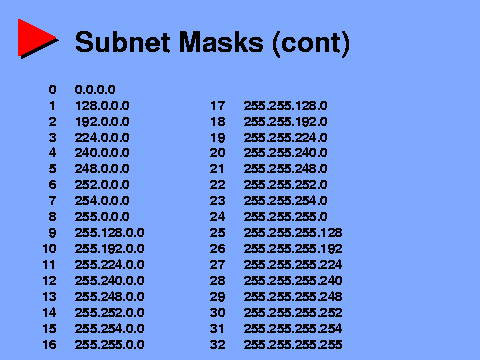Connected: An Internet Encyclopedia

Up: Connected: An Internet Encyclopedia
Up: Programmed Instruction Course
Up: Subnetting and CIDR
Next: Quiz 3Subnet Masks (cont) Just as there are nine possible eight bit numbers that meet the requirements for a subnet mask, so there are only thirty three such thirty two bits numbers. These numbers are shown in this diagram, written in dotted quad notation, and with the corresponding prefix length (the number of one bits in the mask) beside them.

There are no other valid subnet masks of any kind

Again, I suggest you familiarize yourself with this chart. Memorization is not necessary, as this will come with time, but you should be able, with pencil and paper, to convert any prefix length or subnet mask back and forth between the two forms.

For example, a prefix length of 19 corresponds to a subnet mask of 255.255.224.0. Why? Write out 19 one bits, pad them with enough zeros to form a thirty two bit subnet mask (32 - 19 = 13 zeros), and then divide them into four groups of eight bits each:

11111111 11111111 11100000 00000000

Convert each byte to decimal and you get 255.255.244.0

If you are having difficulty seeing the relationship between these numbers, I again suggest you review the Binary Arithmetic page, as well as the last few pages.

Next: Quiz 3

Connected: An Internet Encyclopedia